Documentation

## Catalog of Model Interconnections

Each type of block diagram connection corresponds to a model interconnection command or arithmetic expression. The following tables summarize the block diagram connections with the corresponding interconnection command and arithmetic expression.

### Model Interconnection Commands

Block Diagram ConnectionCommandArithmetic Expression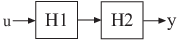`series``(H1,H2)``H2*H1`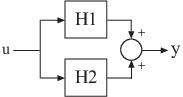`parallel``(H1,H2)``H1+H2`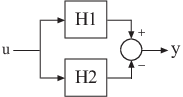`parallel``(H1,-H2)``H1-H2`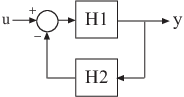`feedback``(H1,H2)``H1/(1+H2*H1)` (not recommended)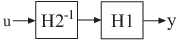N/A`H1/H2` (division)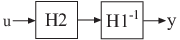N/A`H1\H2` (left division)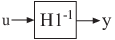`inv(H1)`N/A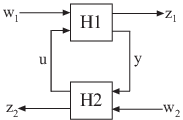`lft``(H1,H2,nu,ny)` N/A

### Arithmetic Operations

You can apply almost all arithmetic operations to dynamic system models, including those shown below.

Operation

Description

`+`

`-`

Subtraction

`*`

Multiplication

`.*`

Element-by-element multiplication

`/`

Right matrix divide

`\`

Left matrix divide

`inv`

Matrix inversion

`'`

Conjugate transposition. See `ctranspose`.

`.'`

Transposition

`^`

Powers of a dynamic system model, as in the following syntax for creating transfer functions:

```s = tf('s'); G = 25/(s^2 + 10*s + 25);```

In some cases, you might obtain better results using model interconnection commands, such as `feedback` or `connect`, instead of model arithmetic. For example, the command `T = feedback(H1,H2)` returns better results than the algebraic expression `T = H1/(1+H2*H1)`. The latter expression duplicates the poles of `H1`, which inflates the model order and might lead to computational inaccuracy.